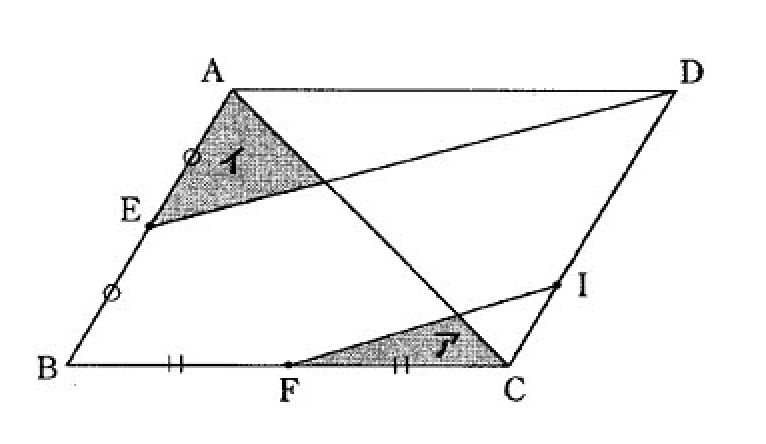# Any Parallelogram Will Work Too?

Geometry Level 2Above shows a parallellogram $ABCD$ with $E$ and $F$ as the midpoints of the straight lines $AB$ and $BC$ respectively. And straight lines $ED$ and $FI$ are parallel to each other.

If ア is $3\text{ cm}^2$, what's the area of イ?

×